#Interactive Real Analysis

Next | Previous | Glossary | Map

## 8.4. Taylor Series

### Proposition 8.4.15: Taylor Series for the Natural Log

ln(1+x) = x - 1/2 x2 + 1/3 x3 - 1/4 x4 + .... =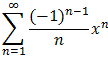for -1 < x1
Note that the series also converges for x = 1.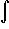1/1+x dx =1/1-(-x) dx =
=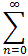(-x)n dx =(-x)n dx =
=(-1)n/n+1 xn+1 =where the computation is valid for -1 < x < 1. It remains to prove the statement for x = 1. But this will be a perfect application of Abel's Limit theorem:converges for |x| < 1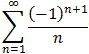converges as the alternating harmonic series

Therefore

ln(2) =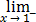ln(1+x) ==according to Abel's Limit theorem.### Fun Facts:

• ln(2) = 1 - 1/2 + 1/3 - 1/4 + ... (but convergence is slow)

This fact has just been proven as an application of Abel's Limit theorem.

Alt. Harmonic Series approaching ln(2)=0.6931472
Next | Previous | Glossary | Map